# Single Phase Voltage Source inverter simulation in Simulink

Single Phase Voltage Source Inverter with Simulink Model: In last article I have posted about forward converter design using simlink Single phase voltage source inverter is a voltage source inverter that inverts the dc voltage into square wave ac or sine wave ac voltages. There are two types of voltage inverters are currently used in market, first one is full bridge voltage source inverter which consists of four switches, IGBTs, or MOSFETs and second one is single phase voltage source inverter which consists of two switches, IGBTs or MOSFETs. These voltage source inverters have been widely used in single phase UPS, switching power supplies and currently these have been mostly used in high power static power typologies. In this article, we would explain how we can make single phase voltage source inverter as well as how we choose the components with the help of MATLAB Simulink model. First, we will explain how we can make a new model in MATLAB. For making a new model, just open the MATLAB and click on Simulink library. After clicking on Simulink library, a new page would be opened that is called a Simulink library browser shown in figure 1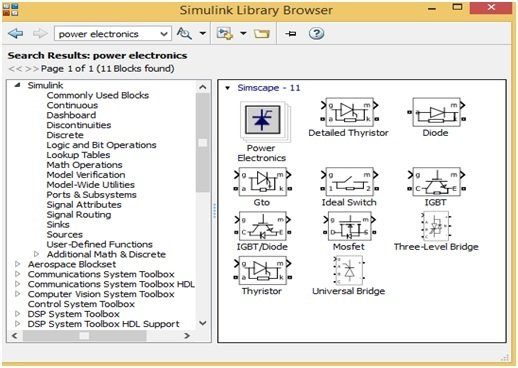This Simulink library browser have different options on their Manu bar just click on new model then model page would be opened shown in figure 2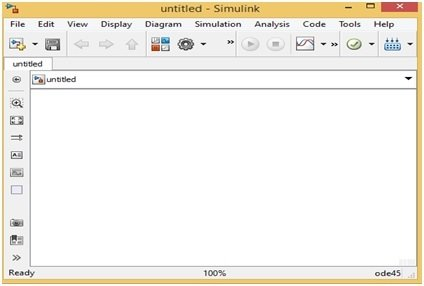On this Simulink new model, we can make our new circuit and then can run or simulate as we want. For choosing the power electronic components, just write the power electronics word on search Manu bar of Simulink library browser then a new page would be opened which have all the power electronics components blocks shown in figure 3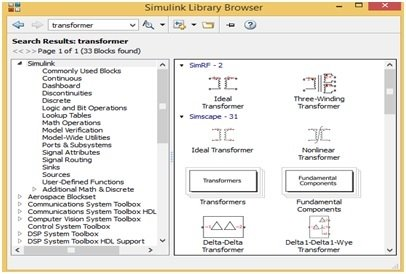Figure 3 Power Electronics Components Blocks

For inserting these blocks on a new model just drags these blocks on a new model or right click on every block and then click on Add block to a new model then this block will automatically copy on a new model. So, we can add these blocks to a new model easily. Similarly, for searching oscilloscope block and display blocks just write the word sink on search Manu bar of Simulink library browser then all block would be opened on a new page shown in figure 4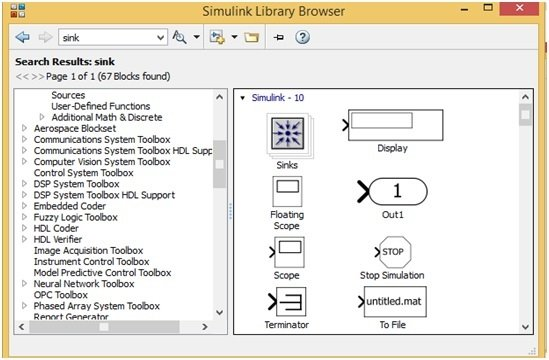Figure 4 Oscilloscope and Display Blocks

Similarly, we search and drag all the other components blocks such as pulse generator, logic gate, capacitor, resistor etc.  and then joint them according to the desired circuit. Now we have made a single-phase voltage source inverter with the help of MATLAB Simulink library shown in figure 5. This circuit have made according to above procedure.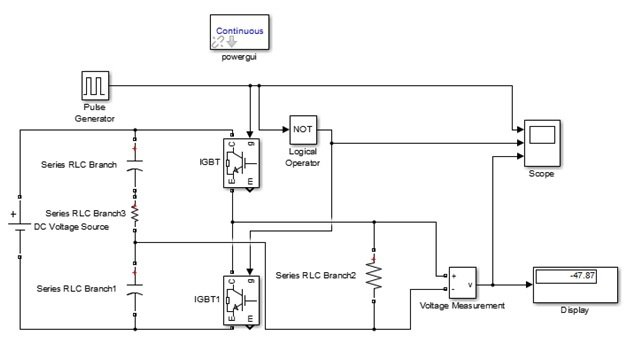Figure 5 Single Phase Voltage Source Inverter with Simulink Model

We have made this single-phase voltage source inverter with Simulink model with the help of two IGBTs, two 50μF capacitors,100V dc voltage supply and one not gate logic operator. One-ohm resistor is attached between two capacitors because the MATLAB simulation not to allow to simulate this circuit without this resistor but in hardware there is no need to joint this resistor. 10Ω resistor is used as an output load. We have run or simulate this circuit at 50% duty cycle. The duty cycle is set by double click on pulse generator and then set according to the figure 6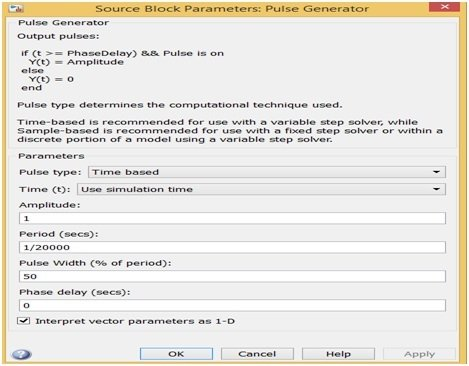Figure 6 Duty Cycle Setting

Because, both IGBTs switches should not on at same time therefore one gate pulse is directly connecting with one IGBTs switch and one gate pulse is connecting with other IGBTs switch after not gate. In other words, this circuit woks in complementary switching. When we simulate this circuit at 50% duty cycle then output voltage would half of the supply voltages means it would 50V shown in figure 7 display.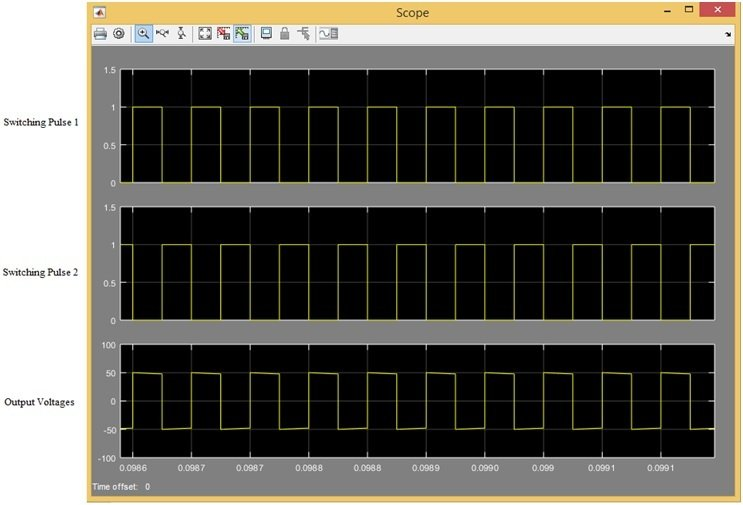Figure 7 Switching Pulse 1, Switching Pulse 2 and Output Voltages